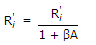Electronics and Communication Engineering - Electronic Devices and Circuits

21.

Recombination produces new electron-hole pairs

 A. True B. False

Explanation:

Due to recombination the number of electron-hole pairs is reduced.

22.

An amplifier without feedback has a voltage gain of 50, input resistance of 1 kΩ and output resistance of 2.5 kΩ. The input resistance of the current shunt -ve feedback amplifier using the above amplifier with a feedback factor of 0.2 is

 A. 1/11 kΩ B. 1/5 kΩ C. 5 kW D. 11 kW

Explanation:

Input Resistance with feedback for current shunt,.

23.

As compared to an ordinary semiconductor diode, a Schottky diode

 A. has lower cut in voltage B. has higher cut in voltage C. lower reverse saturation current D. both (b) and (c)

Explanation:

Cut in voltage in Schottky diode is about 0.3 V as compared to 0.7 V in ordinary semiconductor diode.

24.

Assertion (A): When a high reverse voltage is applied to a p-n junction the diode breaks down.

Reason (R): High reverse voltage causes Avalanche effect.

 A. Both A and R are true and R is correct explanation of A B. Both A and R are true but R is not a correct explanation of A C. A is true but R is false D. A is false but R is true

Explanation:

Avalanche breakdown occurs at high reverse voltage.

25.

As compared to an ordinary semiconductor diode, a Schottky diode

 A. has higher reverse saturation current B. has higher reverse saturation current and higher cut in voltage C. has higher reverse saturation current and lower cut in voltage D. has lower reverse saturation current and lower cut in voltage